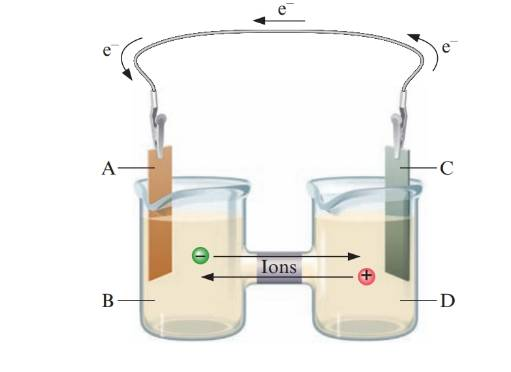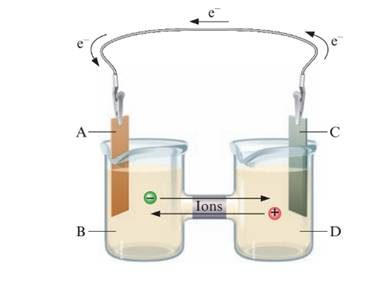Chapter 18, Problem 9ALQ### Introductory Chemistry: A Foundati...

9th Edition
Steven S. Zumdahl + 1 other
ISBN: 9781337399425

#### Solutions

Chapter
Section### Introductory Chemistry: A Foundati...

9th Edition
Steven S. Zumdahl + 1 other
ISBN: 9781337399425
Textbook Problem
6 views

# Label the following parts of the galvanic cell. anode cathode reducing agent oxidizing agentInterpretation Introduction

Interpretation:

The given parts should be labelled in the galvanic cell:Concept Introduction:

The oxidation and reduction can be identified by change in oxidation state. If oxidation state of atom of an element increases, it undergoes oxidation and if it decreases, it undergoes reduction.

The general oxidation and reduction half reactions are as follows:

AsA+aq+e

Here, As undergoes oxidation to form A+aq with release of 1 electron. The oxidation state of A increases from 0 to 1.

Similarly,

B+aq+eBs

Here, B+aq undergoes reduction to form Bs with addition of 1 electron. The oxidation state of A decreases from 1 to 0.

Explanation

The substance that reduces others and itself get oxidised is known as reducing agent and the substance that oxidizes others and itself get reduced is known as oxidizing agent.

Since, oxidation is loss of electrons, electrons are lost from solution D to solution B thus, solution D is where oxidation takes place and it is a reducing agent...

### Still sussing out bartleby?

Check out a sample textbook solution.

See a sample solution

#### The Solution to Your Study Problems

Bartleby provides explanations to thousands of textbook problems written by our experts, many with advanced degrees!

Get Started